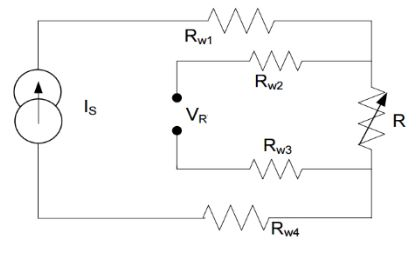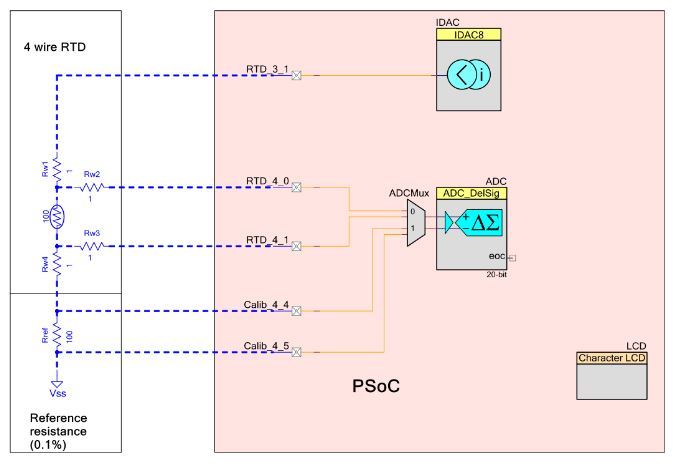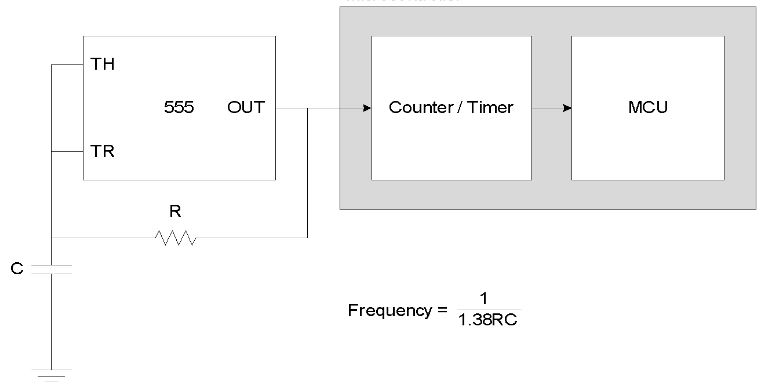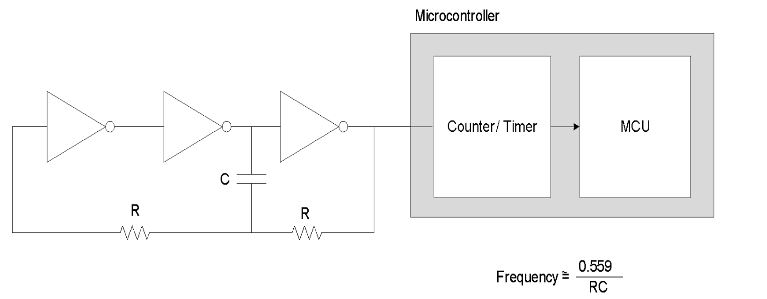# Customised Solution for Measurements: Building a Personal Multimeter (Part-2)

0
3673
Advertisement

Once we are done measuring the voltage and current, let’s take a look at the other measurable parameters that can be handled by a DMM.

Resistance Measurement: There are several methods to measure resistance. The four wire measurement is the most useful as it greatly reduces the error due to wire resistances. In this method, a known constant current is passed through the unknown resistor R. The voltage across it is measured using a separate sensing path.

Advertisement

The separate sensing path ensures that the voltage drop across the wire resistances, Rw1 and Rw4, does not affect the voltage measured. There is little voltage drop across resistances Rw2 and Rw3, which are in the ADC measurement path, because there is negligible current flow into the high-input impedance terminals of the ADC. The unknown resistor in this method is given by the equation R=V/I.Fig 6: Four wire measurement of unknown resistance

To find the unknown resistance, the current source and the ADC measuring the voltage must be accurate. Specifically, the current source and the ADC should be free from offset, gain and non-linearity errors. Even a small error in voltage measurement can result in a large temperature error at higher temperatures. To overcome the gain/offset error caused by the ADC and the current DAC (IDAC), a reference resistor can be added to the design.Fig 7: Unknown resistance measurement using PSoC

Capacitance measurement: DMM uses multiple methods for capacitance measurement. Some of them have been mentioned below.

RC Oscillator Method: In this method, the capacitance to be measured is made part of an RC oscillator and the frequency of the RC oscillator varies with change in capacitance. Figure -8 shows an RC oscillator using 555 timer and Figure-9 shows an RC oscillator using inverters.Fig 8: RC Oscillator using 555 TimerFig 9: RC Oscillator using Inverters

The output of the oscillator can then be fed to a counter to count the number of cycles in a second to measure the frequency and calculate the capacitance using this value.

Alternatively, the oscillator output can be connected to a timer and period can be measured using capture functionality of the timer. Capacitance can be calculated using this value. This method requires external components to construct the RC oscillator and also the oscillator frequency is sensitive to power supply variations.

Advertisement
1
2
3
SHARE